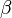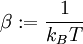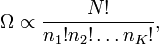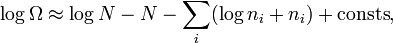Inverse temperature

(diff) ← Older revision | Latest revision (diff) | Newer revision → (diff)

It is often convenient to define a dimensionless inverse temperature,$\beta$:$\beta := \frac{1}{k_BT}$

This notation likely comes from its origin as a Lagrangian multiplier, for which Greek letters are customarily written.

Indeed, it shown in Ref. 1 that this is the way it enters. The task is to maximize number of ways $N$ particles may be asigned to $K$ space-momentum cells. Introducing the partition function:$\Omega\propto\frac{N!}{n_1! n_2! \ldots n_K!} ,$

one could maximize its logarithm (a monotonous function):$\log \Omega \approx \log N -N - \sum_ i ( \log n_i + n_i) + \mathrm{consts} ,$

where Stirling's approximation for large numbers has been used.